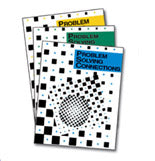# Problem Solving Connections## The best way to systematically develop the key problem solving strategies all students need!

Model the strategies that are essential for solving challenging process problems.

Help all of your students develop the fundamental strategies that good problem solvers need. Problem Solving Connections raises student math scores with systematic problem solving strategies and models each strategy with easy-to-implement techniques. Clear explanations in each lesson minimize teacher preparation time, while practice in "explaining the solution" prepares students for newer assessments.

### What it is...

Problem Solving Connections is an instructional program for grades one through six that systematically develops the fundamental strategies needed for solving challenging problems. More than 25 complex problems in each unit provide opportunities for strategy application in a variety of contexts. The structure of the program is unique in that concepts and strategies are introduced and reviewed in a spiral fashion so that students can enter the program at any level. Problem Solving Connections provides real-life applications to all curricular areas, as well as opportunities to discuss and extend each strategy and to create original problems.

### What it does...

Problem Solving Connections helps students excel on the research-based NCTM Standards. It raises student math scores and prepares students for the newer assessments through practice in "explaining the solution."

Problem Solving Connections:

• Teaches problem solving as a set of strategies to apply on content from across the curriculum
• Models not only how, but also when and why to apply each strategy
• Builds students' higher-level skills in verbalizing, explaining, and justifying their solutions
• Incorporates manipulatives, calculators, and technology as appropriate

### How it works...

Problem Solving Connections incorporates both challenging problems and instruction in the strategies necessary for solving them. Each grade level provides more than two hundred process problems. Every unit develops and applies one of ten strategies that research indicates lead to accelerated problem solving abilities. Lessons within the unit systematically introduce, model, and expand student abilities to use and explain each strategy:

• Estimate
• Act It Out
• Make a Model
• Work Backwards
• Draw a Picture or Diagram
• Make a List/Table/Chart
• Look for a Pattern
• Guess and Check
• Use Logical Reasoning
• Set Up an Equation

### How it works for you...

Problem Solving Connections minimizes teacher preparation time by using clear explanations in each lesson – introducing and modeling each strategy with easy-to-implement techniques. Also included are discussion questions to help students internalize the strategies and apply them in new situations, unit assessments, and cumulative reviews.

Classroom Sets include 1 Teacher Manual and 30 Student Books.
\$270.00

Student Books: each unit focuses on one problem solving strategy, and systematically introduce, model, and expand student abilities to use each strategy.
\$7.00

Teacher Manuals are designed for both teacher generalists and math specialists, offering classroom-tested lesson plans for teaching each strategy.
\$60.00

* Also available in Spanish (Please call 800.225.3214 to order by phone.)

Grade 1 - Red Level

Operational Skills: Addition

Units:
1. Telling Time
2. Working with Money
3. Working with Place Value
4. Working with Graphs
5. Exploring Geometry
6. Working with Fractions

Grade 2 - Green Level

Operational Skills: Addition, Subtraction

Units:
1. Telling Time
2. Working with Money
3. Working with Place Value
4. Working with Graphs
5. Exploring Geometry
6. Working with Fractions

Grade 3 - Orange Level

Operational Skills: Addition, Subtraction, Numeration

Units:
1. Introduction to Problem Solving
2. Supporting Strategies
3. Guess and Check
4. Draw a Picture
5. Make a List
6. Look for a Pattern

Grade 4 - Blue Level

Operational Skills: Addition, Subtraction, Numeration, Multiplication

Units:
1. Supporting Strategies
2. Draw a Picture
3. Make a List, Table, or Chart
4. Look for a Pattern
5. Guess and Check
6. Use Logical Reasoning

Grade 5 - Tan Level

Operational Skills: Addition, Subtraction, Numeration, Multiplication, Division

Units:
1. Supporting Strategies
2. Draw a Picture or Diagram
3. Make a List, Table, or Chart
4. Look for a Pattern
5. Guess and Check
6. Use Logical Reasoning

Grade 6 - Gold Level

Operational Skills: Addition, Subtraction, Numeration, Multiplication, Division, Fractions

Units:
1. Supporting Strategies
2. Draw a Picture or Diagram
3. Make a List, Table, or Chart
4. Guess and Check
5. Use Logical Reasoning
6. Set Up an Equation